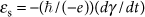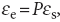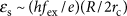Thank you for visiting nature.com. You are using a browser version with limited support for CSS. To obtain the best experience, we recommend you use a more up to date browser (or turn off compatibility mode in Internet Explorer). In the meantime, to ensure continued support, we are displaying the site without styles and JavaScript.

# Spin-motive force due to a gyrating magnetic vortex

## Abstract

A change of magnetic flux through a circuit induces an electromotive force. By analogy, a recently predicted force that results from the motion of non-uniform spin structures has been termed the spin-motive force. Although recent experiments seem to confirm its presence, a direct signature of the spin-motive force has remained elusive. Here we report the observation of a real-time spin-motive force produced by the gyration of a magnetic vortex core. We find a good agreement between the experimental results, theory and micromagnetic simulations, which taken as a whole provide strong evidence in favour of a spin-motive force.

## Introduction

According to theory, motive forces are described by a generalized Faraday law:, where γ, ħ, and e are the Berry phase1, Plank's constant divided by 2π, and the electron's charge, respectively. When γ is the Aharonov–Bohm phase2, this expression gives rise the conventional Faraday law. However, including the spin-Berry phase γs, in the expression gives rise to a spin-motive force (SMF)3,4, which has an opposite sense for the majority/minority electrons of a ferromagnet. Because of this spin imbalance, this effect can be electrically detected in ferromagnets as an effective electromotive force (EMF)where P is the average spin polarization of conduction electrons. The non-coplanar spin structure is responsible for the spin Berry phase γs. We note that the SMF signals arising in a single material are driven by an AC magnetic field, in contrast to the spin voltaic effect5,6, which is realized in a junction system with the circularly polarized light. Recent experiments involving magnetic domain wall propagation, and magnetization reversal of nano-particles embedded in a magnetic tunnel junction have suggested the existence of the SMF7,8. In these experiments, however, the signals were detected at a point far away from the spin texture, and the measuring time was much longer than the characteristic timescale of the system's spin dynamics. Thus, investigating the dynamical nature of the SMF by directly probing the local spin structure is particularly pertinent given the recent controversy concerning the evidence in favour of the SMF9.

A magnetic vortex has a core region with a magnetization normal to the plane of the disc (Fig. 1a–c)10,11, forming such a non-coplanar structure. A time dependence of γs is introduced by the spin dynamics, induced by applying an external in-plane AC magnetic field of frequency fex close to fr for resonance12. When the core passes through the region between the two terminals shown in Fig. 1d, it will induce a SMF that is proportional to the core velocity v (refs 13,14). More quantitatively, it can be estimated as, where fex is the driving frequency, R the radius of gyration (that is, ν=2π fexR) and 2rc the core diameter. At resonance, fex~fr, the SMF is a maximum, reflecting the maxima of R and v. In this Article, we verify this estimate both by experiment and by micromagnetic simulations.Figure 1: Spinmotive force induced by a gyration motion of magnetic vortex and experimental setup.

## Results

### Sample structure and measurement setup

We prepared devices designed specifically for the observation of the SMF induced by the core gyrational motion. The device structure and the measurement configuration are shown schematically in Fig. 1d. A ferromagnetic micro-disc, 4.2 μm in diameter, is made from a 40nm-thick permalloy (Ni80Fe20) film. The aspect ratio is such that a vortex spin structure is formed with its core at the centre of the disc. Below the disc, a copper coplanar waveguide (CWG) is formed, and between the disc and this guide is a SiO2 electrical insulator layer. Two gold electrode pillars, with different diameters (200 and 600 nm), are asymmetrically placed on the disc to detect the SMF signal. The distance between the smaller pillar A and the centre of the disc is 400 nm. The larger pillar B is placed near the edge of the disc (see Methods). An AC current with radio frequency (rf) (50–100 MHz) is injected into the CWG by a signal generator. The applied field (Hrf) is along the in-plane direction to excite the gyrational motion of the core. The induced SMF voltage between the pillars is amplified and measured using a digital oscilloscope (see Methods for details of the measurement). For each measurement, the signal is averaged over more than 60,000 acquisitions.

### Detection of SMF

The resulting signal comprises not only the average of the SMF, but also the conventional EMF induced in the device circuit by the time-varying magnetic flux due to Hrf. To extract the SMF signal, the conventional EMF is measured separately for the same disc in the uniform magnetization state obtained by applying a DC external magnetic field (400 Oe) along the in-plane direction (see Methods)15. The periodic SMF obtained by subtracting the EMF has amplitude ~1 μV for an Hrf=1.3 Oe with frequency (fex) of 80 MHz, as shown in Fig. 2a. The EMF due to a time-varying magnetic flux, produced by the gyrating vortex, has been numerically calculated and is an order of magnitude smaller than the signal amplitude observed here (see Methods).

The experimental results are compared with the SMF calculated in the two-dimensional micromagnetic simulation with parameters appropriate for NiFe. Figure 2b shows the SMF signal simulated for an applied field with amplitude Hrf=1.25 Oe and frequency fex=80 MHz, the latter being close to the resonant frequency (fr). The simulated SMF voltage is defined as the difference in the electrochemical potentials between the two electrode pillars placed as in the experiment. The calculated amplitude of ~1 μV is in good agreement with the measured one. Moreover, the shape of the experimental signal, shown in Fig. 2a, with its sharp negative periodic spikes indicating the close approach of the core to the pillar A, is successfully reproduced in the simulation.

### Excitation frequency dependence of the amplitude of SMF

Figure 2a,b displays the raw data. When comparing the frequency dependence of the experimental and simulated amplitudes, it should be borne in mind that the amplifier has a cutoff frequency of about 100 MHz. Namely, the amplitudes and phases of the measured harmonic signals do not reflect those of the original signals. The fundamental signal is determined using a fast Fourier transform filter (see Methods). The resulting experimental SMF amplitude as a function of frequency fex of the applied field, for an amplitude Hrf=1.0 Oe is shown in Fig. 3a. A peak is seen at about fr (~80 MHz), in good agreement with the experimental fr. The simulated SMF amplitude, for Hrf=1.0 Oe (Fig. 3b), has a peak at fr (85 MHz). At fr, because the radius R of the core gyration is greatest, the core orbit is closest to the pillar A.Figure 3: Excitation frequency (fex) dependence of the amplitude of the spinmotive force.

### Excitation frequency dependence of the amplitude of SMF

Finally, we discuss the Hrf dependence of the SMF amplitude. Figure 4a shows the experimental SMF amplitude as a function of Hrf for a fixed fex=80 MHz. Below ~1.6 Oe, the amplitude increases monotonically with Hrf, as expected, because the radius and velocity of the core motion increases. The abrupt drop at ~1.7 Oe is attributed to the onset of random switching of the core polarity16. The magnetization in the core is perpendicular to the disc plane and can assume two different directions or polarities (up or down). It is known that when the core velocity exceeds a certain value (~250–300 m s−1)17, the switching of the polarity becomes more frequent. Theory also predicts, for the current placement of the electrodes relative to the direction of the applied field, that there is no core polarity phase difference of the steady-state signals. (There would be a 90° phase shift if the pillar configuration is rotated by 90° (refs 12,18).) However, core switching is experimentally known to occur on a timescale that is short compared with the period of oscillations and results in a sudden phase that is shift. The resulting transient should exist for α−1 periods. It follows that when the probability of a reversal approaches unity the signal is strongly suppressed, because our results correspond to an average over ~60,000 acquisitions.

The effect of this core reversal can also be seen in the frequency (fex) dependence of the SMF amplitude. The data for Hrf=1.3, 1.6 and 2.1 Oe are shown in Fig. 4b–d. Dips are seen at around fr, whereas there was no discernible dip for Hrf=1.0 Oe (Fig. 3a). For Hrf=1.3 Oe, there is a narrow dip just below 80 MHz, implying a frequent core reversal at this field and frequency. The dip becomes wider as Hrf is increased to 1.6 Oe and then to 2.1 Oe, which is also consistent with the idea that the critical velocity for core reversal occurring at frequencies further from resonance.

## Discussion

In summary, by comparing theoretical, experimental and numerical results, we have demonstrated that a gyrating vortex core produces a spin-motive force in the direction transverse to its motion. In effect, such a non-uniform spin structure can work as a built-in nano-spin–battery, the output of which is tunable by the magnitude and frequency of the external driving field. Compared with the maximum amplitude that one would naively expect for such a motive force, hfex/e, the observed amplitude is enhanced by a factor proportional to the orbital radius divided by the core diameter.

## Methods

### Measurement setup

The amplifier has a band-pass characteristic with low- and the high-frequency cutoffs of 1 kHz and 100 MHz, respectively. The amplified output voltage (Vout) is monitored by an oscilloscope. A high-pass filter with the cutoff frequency of 27.5 MHz was inserted between the amplifier and the oscilloscope to remove the unwanted DC and low-frequency signals. The maximum voltage gain, between the cutoff frequencies, was 46 dB (the voltage gain Gv ~200) and Gv at 80 MHz was 161. All signals shown here were average of 65,534 acquisitions.

### Ordinary electromotive force induced by RF field

The amplified signal, which includes the ordinary EMF Vout for Hdc=0 and for Hdc=400 Oe are shown in Fig. 5a,b, where Hdc is the DC in-plane external magnetic field. A Hdc of 400 Oe was confirmed to be strong enough to put the disc in a single-domain state15. Fig. 5c shows the difference signal. In Fig. 2a, the amplitude of the difference signal was divided by Gv.

### Numerical calculations

The numerical calculations are performed as described in ref. 13. The Landau–Lifshiz–Gilbert equation is solved using the fourth-order Runge–Kutta method. The NiFe disc contains a magnetic vortex structure of 4.2 μm in diameter, and thickness 40 nm. The saturation magnetization was taken to be 4πMs=1 T, the exchange stiffness A=1×1011 J m−1, and the anisotropy constant K=0, and the Gilbert damping coefficient α=0.01. The system is divided into cells of size 5 nm×5 nm×40 nm. The time evolution is determined using 1 ps time steps. Radius of the core motion as a function of excitation frequency is shown in Fig. 6.

### Ordinary electromotive force induced by vortex dynamics

The magnitude of the usual EMF produced by the gyrating vortex has been estimated by a numerical calculation of the total time-varying magnetic flux and its time derivative. The geometry is that of the real device. The NiFe disc is divided into cells for the numerical calculation. The total magnetic flux density B was first calculated by integrating the magnetic flux densities induced by each cell. To calculate the total magnetic flux, B is integrated over the area surrounded by the electrodes, which are connected to the pillars, as illustrated in Fig. 7, On this basis, the EMF due to the vortex motion was estimated to be at most ~100 nV for fex=80 MHz and Hrf=1.0 Oe.

### Fast Fourier transform (FFT) filter

First, the FFT of the difference signal is obtained. Then, the spectra below 27.5 MHz and above 100 MHz are removed. Next the amplitude is divided by the frequency-dependent gain of the amplifier and the inverse FFT performed. The resulting amplitudes are shown in Figs 3 and 4.

How to cite this article: Tanabe, K. et al. Spin-motive force due to a gyrating magnetic vortex. Nat. Commun. 3:845 doi: 10.1038/ncomms1824 (2012).

## References

1. 1

Berry, M. V. Quantal phase factors accompanying adiabatic changes. Proc. R. Soc. A 392, 45–57 (1984).

2. 2

Aharonov, Y. & Bohm, D. Significance of electromagnetic potentials in the quantum theory. Phys. Rev. 115, 485–491 (1959).

3. 3

Barnes, S. E. & Maekawa, S. Generalization of Faraday's law to include nonconservative spin forces. Phys. Rev. Lett. 98, 246601 (2007).

4. 4

Stern, A. Berry's phase, motive forces, and mesoscopic conductivity. Phys. Rev. Lett. 68, 1022–1025 (1992).

5. 5

Kondo, T., Hayafuji, J.-j. & Munekata, H. Investigation of spin voltaic effect in a p–n heterojunction. Jpn J. Appl. Phys. 45, L663–L665 (2006).

6. 6

Žutić, I., Fabian, J. & Sarma, S. D. Spin-polarized transport in inhomogeneous magnetic semiconductors: theory of magnetic/nonmagnetic p-n Junctions. Phys. Rev. Lett. 88, 066603 (2002).

7. 7

Yang, S. A. et al. Universal electromotive force induced by domain wall motion. Phys. Rev. Lett. 102, 067201 (2009).

8. 8

Hai, P. N., Ohya, S., Tanaka, M., Barnes, S. E. & Maekawa, S. Electromotive force and huge magnetoresistance in magnetic tunnel junctions. Nature 458, 489–492 (2009).

9. 9

Ralph, D. C. The electromotive force of MnAs nanoparticles. Nature 474, E6 (2011).

10. 10

Shinjo, T., Okuno, T., Hassdorf, R., Shigeto, K. & Ono, T. Magnetic vortex core observation in circular dots of permalloy. Science 289, 930–932 (2000).

11. 11

Wachowiak, A. et al. Direct observation of internal spin structure of magnetic vortex cores. Science 298, 577–580 (2002).

12. 12

Novosad, V. et al. Magnetic vortex resonance in patterned ferromagnetic dots. Phys. Rev. B 72, 024455 (2005).

13. 13

Ohe, J.-i. & Maekawa, S. Spin motive force in magnetic nanostructures. J. Appl. Phys. 105, 07C706 (2009).

14. 14

Ohe, J.-i., Barnes, S. E., Lee, H.- W. & Maekawa, S. Electrical measurements of the polarization in a moving magnetic vortex. Appl. Phys. Lett. 95, 123110 (2009).

15. 15

Tanabe, K., Chiba, D. & Ono, T. Electrical detection of magnetic vortex chirality. Jpn. J. Appl. Phys. 49, 078001 (2010).

16. 16

Waeyenberge, B. V. et al. Magnetic vortex core reversal by excitation with short bursts of an alternating field. Nature 444, 461–464 (2006).

17. 17

Lee, K.- S. et al. Universal criterion and phase diagram for switching a magnetic vortex core in soft magnetic nanodots. Phys. Rev. Lett. 101, 267206 (2008).

18. 18

Moon, J.- H. & Lee, K.- J. Spin-motive force caused by vortex gyration in a circular nanodisk with holes. J. Magn. 16, 6–9 (2011).

## Acknowledgements

We thank Fukami, S. and Ishiwata, N. for film deposition. This work was supported in part by Grant-in-Aid for Scientific Research (S) from JSPS, Grant-in-Aid for Scientific Research in Priority Area 'Creation and control of spin current' from MEXT, Grant-in-Aid for JSPS Fellows, and the Asahi Glass Foundation.

## Author information

Authors

### Contributions

K.T. fabricated the device. K.T., D.C. and S.K. set up measurement apparatus and collected all data. J.O., H.K., S.E.B. and S.M. studied theoretically. K.T., D.C., K.K. and T.O. wrote the manuscript. All authors discussed the results.

### Corresponding author

Correspondence to T. Ono.

## Ethics declarations

### Competing interests

The authors declare no competing financial interests.

## Rights and permissions

Reprints and Permissions

Tanabe, K., Chiba, D., Ohe, J. et al. Spin-motive force due to a gyrating magnetic vortex. Nat Commun 3, 845 (2012). https://doi.org/10.1038/ncomms1824

• Accepted:

• Published:

• ### Effect of heating on spin-motive force induced by magnetic resonance

• Kenji Tanabe

Applied Physics Letters (2020)

• ### Observation of spin-motive force in ferrimagnetic GdFeCo alloy films

• Shun Fukuda
• , Hiroyuki Awano
•  & Kenji Tanabe

Applied Physics Letters (2020)

• ### Spinmotive force in the out-of-plane direction generated by spin wave excitations in an exchange-coupled bilayer element

• W. Zhou
• , T. Seki
• , H. Imamura
• , J. Ieda
•  & K. Takanashi

Physical Review B (2019)

• ### Spin-driven electrical power generation at room temperature

• K. Katcko
• , E. Urbain
• , B. Taudul
• , F. Schleicher
• , J. Arabski
• , E. Beaurepaire
• , B. Vileno
• , D. Spor
• , W. Weber
• , D. Lacour
• , S. Boukari
• , M. Hehn
• , M. Alouani
• , J. Fransson
•  & M. Bowen

Communications Physics (2019)

• ### Effective gauge field theory of spintronics

• Gen Tatara

Physica E: Low-dimensional Systems and Nanostructures (2019)